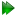Frame order axis permutations

Multiple local minima exist in the optimisation space for the isotropic and pseudo-elliptic cone frame order models. In the case of the pseudo-ellipse, the eigenframes at each minimum are identical, however the θx, θx, and σmax half-angles are permuted. Because of the constraint θxθy in the pseudo-ellipse model, there are exactly three local minima (out of 6 possible permutations). In the isotropic cone, the θxθy condition collapses this to two. The multiple minima correspond to permutations of the motional system - the eigenframe x, y and z-axes as well as the cone opening angles θx, θy, and σmax associated with these axes. But as the mechanics of the cone angles is not identical to that of the torsion angle, only one of the three local minima is the global minimum.

As the minfx library used in the frame order analysis currently only implements local optimisation algorithms, and because a global optimiser cannot be guaranteed to converge to the correct minima, a different approach is required:

• Optimise to one solution.
• Duplicate the data pipe for the model as permutation A'.
• Permute the axes and amplitude parameters to jump from one local minimum to the other.
• Optimise the new permuted model, as the permuted parameters will not be exactly at the minimum.
• Repeat for the remaining permutation B' solution (only for the pseudo-ellipse models).

These steps have been incorporated into the automated analysis protocol.

The permutation step has been implemented as the frame_order.permute_axes user function. It is complicated by the fact that θx is defined as a rotation about the y-axis and θy is about the x-axis. See table 12.1 on pagefor the pseudo-ellipse model permutations. These are also illustrated in figure 12.2 on page.

For the isotropic cone model, the same permutations exist but with some differences:

• The x and y axes are not defined in the x-y plane, therefore there are only two permutations (the first solution and `permutation A').
• Any axis in the x-y plane can be used for the permutation, however different axes will result in different χ2 values.
• As θxθy, the condition θxσmaxθy can only exist if the torsion and cone angles are identical.
• Permutations A and B create identical cones as the x and y axes are equivalent.

The new isotropic cone angle is defined as

 θ' =. (12.78)

The isotropic cone axis permutations are shown in figure 12.3 on page.The relax user manual (PDF), created 2020-08-26.